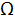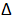# What is RAB

1.  15

2.  30

3.  45

4.  50

4

30Explanation :
No Explanation available for this question

# Stability w.r.t β or S(β) is denoted as

1.  IC/ICO

2.  IC/VBE

3.  IC/β

4.  VBE/IC

4IC/β

Explanation :
No Explanation available for this question

# In emitter bias configuration stability will tend to unity if

1.   (RB/RE)>>(B+1)

2.  (RB/RE)>>1

3.  (RB/RE)

4.  None of these

4

(RB/RE)<<1

Explanation :
No Explanation available for this question

# In Ge at T=400k, the intrinsic concentration is

1.  26.3*1014cm-3

2.  18.4*1014cm-3

3.  8.5*1014cm-3

4.  3.6*1014cm-3

4

8.5*1014cm-3

Explanation :
No Explanation available for this question

# In a hybrid equivalent transistor circuit, hre stands for

1.   Open circuit reverse transfer voltage ratio

2.  Short circuit reverse transfer voltage ratio

3.  Open circuit reverse transfer current ratio

4.  None of these

4

Open circuit reverse transfer voltage ratio

Explanation :
No Explanation available for this question

# Which one of the following statement is correct

1.  The Fermi level in the two materials are at the same energy level irrespective of the number of free electron in copper & silicon

2.  The Fermi Level of copper is of the highest energy level compared to that of silicon as copper has more free electrons

3.  The Fermi Level of Silicon is of the highest energy level compared to that of Copper as silicon has more free electrons

4.  Copper and silicon, being in thermal equilibrium , will have the same specific heat and Fermi levels have no relation to thermal equilibrium

4

The Fermi level in the two materials are at the same energy level irrespective of the number of free electron in copper & silicon

Explanation :
No Explanation available for this question

# What is i1 the given circuit

1.  0.5A

2.  1A

3.  1.5A

4.  3.0A

4

1.5A

Explanation :
No Explanation available for this question

# In a h-parameter transistor model unit of hoe is

1.   Ohms

2.  Volts/sec

3.  Siemens

4.  None of these

4

Siemens

Explanation :
No Explanation available for this question

# Current I in 8resistor is -1A then what is value of V

1.  10V

2.  12V

3.  -10V

4.  None of these

4

12V

Explanation :
No Explanation available for this question

# For a crystal, which one of the following Statement is Correct

1.  The total number of positive wave function in any energy band is equal to the number of unit cells

2.  The total number of positive wave function in any energy band is equal to the twice the number of unit cells as per Pauli principle

3.  The total number of positive wave function in any energy band is equal to the total number of free electrons in the band

4.  The number of positive wave function in any energy band is independent of the number of unit cells

4